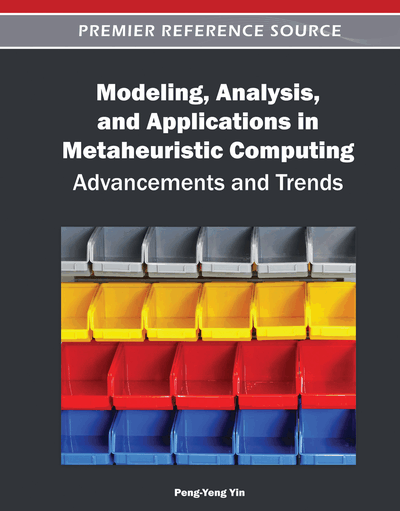# Metaheuristic Search with Inequalities and Target Objectives for Mixed Binary Optimization Part I: Exploiting Proximity

Fred Glover (OptTek Systems, Inc., USA) and Saïd Hanafi (Universite de Valenciennes, France)
Copyright: © 2012 |Pages: 16
DOI: 10.4018/978-1-4666-0270-0.ch001
OnDemand PDF Download:
Available
\$37.50
No Current Special Offers

## Abstract

Recent adaptive memory and evolutionary metaheuristics for mixed integer programming have included proposals for introducing inequalities and target objectives to guide the search. These guidance approaches are useful in intensification and diversification strategies related to fixing subsets of variables at particular values, and in strategies that use linear programming to generate trial solutions whose variables are induced to receive integer values. In Part I (the present paper), we show how to improve such approaches by new inequalities that dominate those previously proposed and by associated target objectives that underlie the creation of both inequalities and trial solutions. Part I focuses on exploiting inequalities in target solution strategies by including partial vectors and more general target objectives. We also propose procedures for generating target objectives and solutions by exploiting proximity in original space or projected space. Part II of this study (to appear in a subsequent issue) focuses on supplementary linear programming models that exploit the new inequalities for intensification and diversification, and introduce additional inequalities from sets of elite solutions that enlarge the scope of these models. Part II indicates more advanced approaches for generating the target objective based on exploiting the mutually reinforcing notions of reaction and resistance. Our work in the concluding segment, building on the foundation laid in Part I, examines ways our framework can be exploited in generating target objectives, employing both older adaptive memory ideas of tabu search and newer ones proposed here for the first time.
Chapter Preview
Top

## 1. Notation And Problem Formulation

We represent the mixed integer programming problem in the form

We assume that Ax + Dyb includes the inequalities Ujxj ≥ 0, jN = {1, …, N}, where some components of Uj may be infinite. The linear programming relaxation of (MIP) that results by dropping the integer requirement on x is denoted by (LP). We further assume Ax + Dyb includes an objective function constraint xoUo, where the bound Uo is manipulated as part of a search strategy for solving (MIP), subject to maintaining Uo < xo*, where xo* is the xo value for the currently best known solution x* to (MIP).

The current paper focuses on the zero-one version of (MIP) denoted by (MIP:0-1), in which Uj = 1 for all jN. We refer to the LP relaxation of (MIP:0-1) likewise as (LP), since the identity of (LP) will be clear from the context,

In the following we make reference to two types of search strategies: those that fix subsets of variables to particular values within approaches for exploiting strongly determined and consistent variables, and those that make use of solution targeting procedures. As developed here, the latter solve a linear programming problem LP(x′,c′)1 that includes the constraints of (LP) (and additional bounding constraints in the general (MIP) case) while replacing the objective function xo by a linear function vo = cx. The vector x′ is called a target solution, and the vector c′ consists of integer coefficients cj′ that seek to induce assignments xj = xj′ for different variables with varying degrees of emphasis.

We adopt the convention that each instance of LP(x′, c′) implicitly includes the (LP) objective of minimizing the function xo = fx + gy as a secondary objective, dominated by the objective of minimizing vo = cx, so that the true objective function consists of minimizing ωo = Mvo + xo, where M is a large positive number. As an alternative to working with ωo in the form specified, it can be advantageous to solve LP(x′,c′) in two stages. The first stage minimizes vo = cx to yield an optimal solution x = x″ (with objective function value vo″ = cx″), and the second stage enforces vo = vo″ to solve the residual problem of minimizing xo = fx + gy.2

A second convention involves an interpretation of the problem constraints. Selected instances of inequalities generated by approaches of the following sections will be understood to be included among the constraints Ax + Dyb of (LP). In our definition of LP(x′, c′) and other linear programs related to (LP), we take the liberty of representing the currently updated form of the constraints Ax + Dyb by the compact representation xX = {x: (x,y) ∈ Z}, recognizing that this involves a slight distortion in view of the fact that we implicitly minimize a function of y as well as x in these linear programs.3

## Complete Chapter List

Search this Book:
Reset